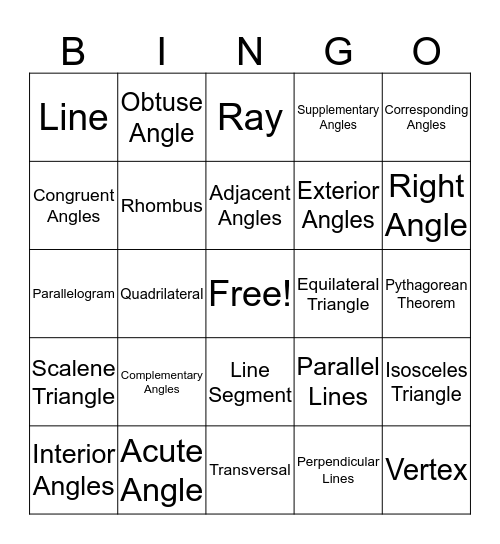# Geometry BingoThis bingo card has a free space and 24 words: Complementary Angles, Acute Angle, Adjacent Angles, Interior Angles, Corresponding Angles, Line, Congruent Angles, Obtuse Angle, Equilateral Triangle, Line Segment, Exterior Angles, Parallelogram, Parallel Lines, Isosceles Triangle, Rhombus, Perpendicular Lines, Pythagorean Theorem, Ray, Right Angle, Quadrilateral, Vertex, Scalene Triangle, Supplementary Angles and Transversal.

## Play Online